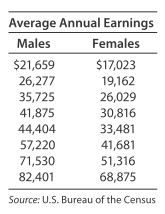# Earnings and gender The data in the table show the average earnings of year-round full-time workers by gender for several different levels of educational attainment. Use linear regression to find the linear equation that is the best for these data, with x representing the earnings for males and y representing the earnings for females. Find and interpret the slope of the linear regression line. If you made a similar linear model with data from 1965, how do you think its slope would compare with the slope found in part (b)? Explain.### Mathematical Applications for the ...

11th Edition
Ronald J. Harshbarger + 1 other
Publisher: Cengage Learning
ISBN: 9781305108042

#### Solutions

Chapter
Section### Mathematical Applications for the ...

11th Edition
Ronald J. Harshbarger + 1 other
Publisher: Cengage Learning
ISBN: 9781305108042
Chapter 14.4, Problem 33E
Textbook Problem
1 views

## Earnings and gender The data in the table show the average earnings of year-round full-time workers by gender for several different levels of educational attainment.Use linear regression to find the linear equation that is the best for these data, with x representing the earnings for males and y representing the earnings for females. Find and interpret the slope of the linear regression line. If you made a similar linear model with data from 1965, how do you think its slope would compare with the slope found in part (b)? Explain.

This textbook solution is under construction.

### Still sussing out bartleby?

Check out a sample textbook solution.

See a sample solution

#### The Solution to Your Study Problems

Bartleby provides explanations to thousands of textbook problems written by our experts, many with advanced degrees!

Get Started